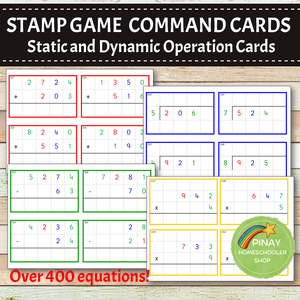Pinay Homeschooler Shop

# Montessori Stamp Game Command Cards BUNDLE

Regular price \$14.00 \$0.00

This Montessori Math BUNDLE has everything you need to present and work on four operations in STAMP GAME. It has over 400+ equations to solve, with two sets each for static and dynamic addition, subtraction, multiplication and division.

WHAT YOU WILL GET:

• 60 static equations, a combination of 2 digits, 3 digits and 4 digits addends
• 60 dynamic equations, a combination of 2 digits, 3 digits and 4 digits addends

⭐️SUBTRACTION (green border)

• 60 static equations, a combination of 2 digits, 3 digits and 4 digits minuend/subtrahend
• 60 dynamic equations, a combination of 2 digits, 3 digits and 4 digits minuend/subtrahend

⭐️MULTIPLICATION (yellow border)

• 32 static equations, a combination of 2 digits, 3 digits and 4 digits multiplicand and 1 digit multiplier
• 60 dynamic equations, a combination of 2 digits, 3 digits and 4 digits multiplicand and 1 digit multiplier

⭐️DIVISION (blue border)

• 60 static equations, a combination of 2 digits, 3 digits and 4 digits dividend and 1 digit divisor
• 60 dynamic equations, a combination of 2 digits, 3 digits and 4 digits dividend and 1 digit divisor

⭐️CONTROL CARDS FOR EACH

⭐️BLANK COLOR CODED BORDER TEMPLATE